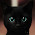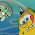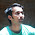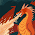## Friday, 27 July 2012

### 00 to 99 Two Digit Decimal Counter via 7 Segment Display Using Verilog

Since I have already made a detailed post regarding 7 segment LED multiplexing, this post is going to be a short one, in which I will only explain the code via comments in code.

Here I am going to make a 2 digit counter that counts from 00 to 99 and then rolls over back to 00. The counter will increment every 0.1 second. The 0.1 second interval is produced by another counter that will produce an enable tick every 0.1 second to increment our main counter.

### How to make a 0.1 second accurate delay in Verilog

We know that the board being used has a 50 MHz clock. So to produce a 0.1 second delay simply multiply the two.. 50Mhz * 0.1 sec = 5000000. So every 5M ticks is equal to 0.1 second. So using the simple log formula ( (x)log(2) = log(5000000) ) we can calculate that a 23 bit wide register will be able to hold a count of 5000000.

The code for this counter is given below:

```module twodigit_onefile(
input clock,
input reset,
output a,
output b,
output c,
output d,
output e,
output f,
output g,
output dp,
output [3:0]an
);

reg [3:0]first; //register for the first digit
reg [3:0]second; //register for the second digit

reg [22:0] delay; //register to produce the 0.1 second delay
wire test;

always @ (posedge clock or posedge reset)
begin
if (reset)
delay <= 0;
else
delay <= delay + 1;
end

assign test = &delay; //AND each bit of delay with itself; test will be high only when all bits of delay are high

always @ (posedge test or posedge reset)
begin
if (reset) begin
first <= 0;
second <= 0;
end
else if (first==4'd9) begin  //x9 reached
first <= 0;
if (second == 4'd9) //99 reached
second <= 0;
else
second <= second + 1;

end
else
first <= first + 1;
end

//Multiplexing circuit below

localparam N = 18;

reg [N-1:0]count;

always @ (posedge clock or posedge reset)
begin
if (reset)
count <= 0;
else
count <= count + 1;
end

reg [6:0]sseg;
reg [3:0]an_temp;
always @ (*)
begin
case(count[N-1:N-2])

2'b00 :
begin
sseg = first;
an_temp = 4'b1110;
end

2'b01:
begin
sseg = second;
an_temp = 4'b1101;
end

2'b10:
begin
sseg = 6'ha; //unknown sent to produce '-'
an_temp = 4'b1011;
end

2'b11:
begin
sseg = 6'ha; //unknown sent to produce '-'
an_temp = 4'b0111;
end
endcase
end
assign an = an_temp;

reg [6:0] sseg_temp;
always @ (*)
begin
case(sseg)
4'd0 : sseg_temp = 7'b1000000; //0
4'd1 : sseg_temp = 7'b1111001; //1
4'd2 : sseg_temp = 7'b0100100; //2
4'd3 : sseg_temp = 7'b0110000; //3
4'd4 : sseg_temp = 7'b0011001; //4
4'd5 : sseg_temp = 7'b0010010; //5
4'd6 : sseg_temp = 7'b0000010; //6
4'd7 : sseg_temp = 7'b1111000; //7
4'd8 : sseg_temp = 7'b0000000; //8
4'd9 : sseg_temp = 7'b0010000; //9
default : sseg_temp = 7'b0111111; //dash
endcase
end
assign {g, f, e, d, c, b, a} = sseg_temp;
assign dp = 1'b1; //we dont need the decimal here so turn all of them off

endmodule
```

The multiplexing circuit has been explained and commended in detail in my previous post here : Verilog LED Multiplexing Circuit.

1.I was trying your code posted above and I get a warning of "Size mismatch between case item and case selector." on the case(sseg) part.
Any help would be greatly appreciated.

1.Hi,

This usually happens when you are trying to write to register used in case(in this case sseg) in two different always blocks.

Please check your code for this error.

The above code was copied here from my xilinx IDE just before making the video demonstration, so I can assure you its error free.

2.Okay, well I copied the code from above directly and put it in my project in Xilinx 13.4 and get that warning in the Place & Route portion.
This is all I am using in my ucf file:
NET "clock" LOC = "B8"; # Bank = 0, Signal name = MCLK
NET "reset" LOC = "M6"; # Bank = 2, Signal name = UCLK

NET "an<3>" LOC = "K14"; # Bank = 1, Signal name = AN3
NET "an<2>" LOC = "M13"; # Bank = 1, Signal name = AN2
NET "an<1>" LOC = "J12"; # Bank = 1, Signal name = AN1
NET "an<0>" LOC = "F12"; # Bank = 1, Signal name = AN0
NET "dp" LOC = "N13"; # Bank = 1, Signal name = DP

3.Honestly if it is a warning and not an error, it can usually be ignored. Also which board are you using, is it the BASYS2? Here is my ucf file, you can try with this:

# Pin assignment for pushbutton switches
NET "reset" LOC = "a7";

# Pin assignment for 7-segment displays
NET "a" LOC = "l14" ;
NET "b" LOC = "h12" ;
NET "c" LOC = "n14" ;
NET "d" LOC = "n11" ;
NET "e" LOC = "p12" ;
NET "f" LOC = "l13" ;
NET "g" LOC = "m12" ;
NET "dp" LOC = "n13" ;

NET "an<3>" LOC = "k14";
NET "an<2>" LOC = "m13";
NET "an<1>" LOC = "j12";
NET "an<0>" LOC = "f12";

# Pin assignment for clock
NET "clock" LOC = "b8";

4.Ah, that fixed it.
Yes, it is with the BASYS2 FPGA.

Thank you so much.

2.Can you help me ?

i am having a problem using FPGA NEXYS 3 Spartan-6 ,

for some reason it only shows number 9 ...

is their problem with code or the assignments or both ?!

another question ... how can i edit the code to make the maximum 10 or 15 for example ?

1.Hello Jack,

The above code has been tested to work on a BASYS2 board. I do not have a NEXYS 3. Most probably you might have to check your ucf file and see if your pin mapping is spot on.

And to change the maximum to 15 or any number can be achieved by editing this code block:

else if (first==4'd9) begin //x9 reached
first <= 0;
if (second == 4'd9) //99 reached
second <= 0;
else
second <= second + 1;

3.Sir I confused why you use this-------assign test = &delay; I mean sir I know you use this here for .1 sec delay but sir why you not use------
if(delay==24'b11111111_11111111_11111111)condition or event
@(delay==24'b11111111_11111111_11111111) whenever delay register is filled with desired bit...........please clearify it specially &delay and why you use wire for test and not reg and why test is just I bit wide and not 23 bit wide like that of delay register....for making it global variable?

1.For "assign test = &delay" I mentioned in comments the reason. The function of & is to logically AND every bit of delay with each other. So test will only be high when ALL the bits of delay are high, otherwise it will be 0 or low.

Yes, you can use the if block you mentioned to achieve the same result, but this was easier and quicker.

test is not inside any always block that is why its a wire and it is not supposed to retain its value outside of the module. Also you cannot use reg with assign.

test is only a flag, it does not have to be 23 bits wide. 1 bit will suffice

4.sir, why are we checking 2 MSB's of counter and then accordingly we are enabling the anodes of display??

1.hello Jyotsana,

I believe you are talking about the counter related to the seven segment multiplexing. I have explained that in detain in my other post here: http://simplefpga.blogspot.co.uk/2012/07/seven-segment-led-multiplexing-circuit.html

I believe you will get both your answers by reading that post.

Regards

5.May i know what is function for the below coding?

reg [6:0]sseg;
reg [3:0]an_temp;
always @ (*)
begin
case(count[N-1:N-2])

2'b00 :
begin
sseg = first;
an_temp = 4'b1110;
end

2'b01:
begin
sseg = second;
an_temp = 4'b1101;
end

2'b10:
begin
sseg = 6'ha; //unknown sent to produce '-'
an_temp = 4'b1011;
end

2'b11:
begin
sseg = 6'ha; //unknown sent to produce '-'
an_temp = 4'b0111;
end
endcase
end

1.Thats the code for the seven segment LED multiplexing circuit. Read more about it here: http://simplefpga.blogspot.co.uk/2012/07/seven-segment-led-multiplexing-circuit.html

6.Hi, I have nexys 3 spartan 6 with 100Mhz bacesclock, I want to increment segment number every 0.5 second so delay register should consists 26 bits since log(0.5*100000000) / log (2) = 25.57, What about the COUNT register? I did not understand how to determinate it's size

1.The method is explained in more detail here: http://simplefpga.blogspot.co.uk/2012/07/to-code-stopwatch-in-verilog.html

7.Hello everybody,

can someone explain me the Pin Assignment for

output [3:0]an

1.Hi Dennis,

The ``output[3:0]an`` is the 4 bit enable signal for multiplexing the four seven segment displays. It has been explained in detail in its own post here: http://simplefpga.blogspot.co.uk/2012/07/seven-segment-led-multiplexing-circuit.html

8.Do you have to have the delay? What is the point in having the delay?

1.The delay is necessary for us, it allows us to see the count. Without the delay the count would increment so fast (at clock speed) we would not be able to see the numbers changing.

9.I want it to work with a push button so that when I press the button it counts by +1. How can I do that ?

1.see my other posts where I have used buttons to control the code. To explain it briefly it should be similar to how we use the reset button, always @ (posedge reset).....

10.hi , i had one problem that i confused
In this sample
case(count[N-1:N-2]) , how does it work ?
why 2'b01 will choose the digit one , 2'b01 choose the digit two

1.Hi, see 7 segment multiplexing explanation for your answer: http://simplefpga.blogspot.co.uk/2012/07/seven-segment-led-multiplexing-circuit.html

11.hi
I have a problem in simulating this example in xilinx IDE
I change value of clock manually but the output just has a X value i don't know why is that.
could u help me?

12.Hello,
i need to add one more logic i.e count from 99 to 00 when an extra input(assume top_down) is on. How can i make changes in the code.
* Note :I want both 00 to 99 and from 99 to 00

1.just subtract in stead of adding, if you want to do both then map two buttons for each task

for example:

always @ (posedge clock or posedge reset)
begin
if (reset)
count <= 99;
else
count <= count - 1;
end

13.Hi, i now want to reverse the numbers from 99 to 00 what changes should be done?

1.always @ (posedge clock or posedge reset)
begin
if (reset)
count <= 99;
else
count <= count - 1;
end

14.I edited your code and i was able to count up to 9999. i edited the code to display number 1234 but i'm not able to get it. Sir can you help me how I should approach to display four digit number eg:1234 with no counting. i removed the delay.

1.if you just need to display the number 1234 then you dont need a counter, just follow this post:https://simplefpga.blogspot.co.uk/2012/07/seven-segment-led-multiplexing-circuit.html

15.Can you please post a vhdl code for this.